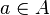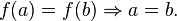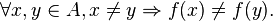# Blog Archives

## Surjection

A function is surjective (onto) if every possible image is mapped to by at least one argument. In other words, every element in the codomain has non-empty preimage. Equivalently, a function is surjective if its image is equal to its codomain. A surjective function is a surjection. The formal definition is the following.

The functionis surjective iff for all, there issuch that f(a) = b.
• A function f : AB is surjective if and only if it is right-invertible, that is, if and only if there is a function g: BA such that f o g = identity function on B. (This statement is equivalent to the axiom of choice.)
• By collapsing all arguments mapping to a given fixed image, every surjection induces a bijection defined on a quotient of its domain. More precisely, every surjection f : AB can be factored as a projection followed by a bijection as follows. Let A/~ be the equivalence classes of A under the following equivalence relation: x ~ y if and only if f(x) = f(y). Equivalently, A/~ is the set of all preimages under f. Let P(~) : AA/~ be the projection map which sends each x in A to its equivalence class [x]~, and let fP : A/~ → B be the well-defined function given by fP([x]~) = f(x). Then f = fP o P(~). A dual factorisation is given for injections above.
• The composition of two surjections is again a surjection, but if g o f is surjective, then it can only be concluded that g is surjective. See the figure at right*.

## Injection

A function is injective (one-to-one) if every possible element of the codomain is mapped to by at most one argument. Equivalently, a function is injective if it maps distinct arguments to distinct images. An injective function is an injection. The formal definition is the following.

The functionis injective iff for all, we have• A function f : AB is injective if and only if A is empty or f is left-invertible; that is, there is a function g : f(A) → A such that g o f = identity function on A. Here f(A) is the image of f.
• Since every function is surjective when its codomain is restricted to its image, every injection induces a bijection onto its image. More precisely, every injection f : AB can be factored as a bijection followed by an inclusion as follows. Let fR : Af(A) be f with codomain restricted to its image, and let i : f(A) → B be the inclusion map from f(A) into B. Then f = i o fR. A dual factorisation is given for surjections below.
• The composition of two injections is again an injection, but if g o f is injective, then it can only be concluded that f is injective. See the figure at right.
• Every embedding is injective.

## Bijection, injection and surjection

In mathematics, injections, surjections and bijections are classes of functions distinguished by the manner in which arguments (input expressions from the domain) and images (output expressions from the codomain) are related or mapped to each other.

A function maps elements from its domain to elements in its codomain.

• A functionis injective (one-to-one) if every element of the codomain is mapped to by at most one element of the domain. Notationally,or, equivalently,An injective function is an injection. Read the rest of this entry

## RANGE

In mathematics, the range of a function refers to either the codomain or the image of the function, depending upon usage. This ambiguity is illustrated by the function f that maps real numbers to real numbers with f(x) = x2. Some books say that range of this function is its codomain, the set of all real numbers, reflecting that the function is real-valued. These books call the actual output of the function the image. This is the current usage for range in computer science. Other books say that the range is the function’s image, the set of non-negative real numbers, reflecting that a number can be the output of this function if and only if it is a non-negative real number. In this case, the larger set containing the range is called the codomain. This usage is more common in modern mathematics. Read the rest of this entry